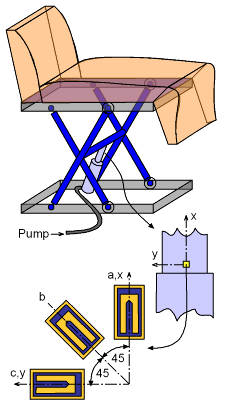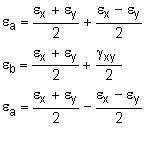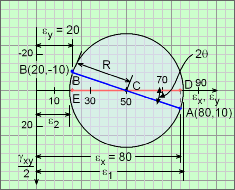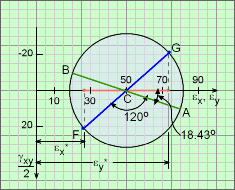Ch 8. Strain Analysis Multimedia Engineering Mechanics PlaneStrain Mohr's Circlefor Strain StrainGages
 Chapter 1. Stress/Strain 2. Torsion 3. Beam Shr/Moment 4. Beam Stresses 5. Beam Deflections 6. Beam-Advanced 7. Stress Analysis 8. Strain Analysis 9. Columns Appendix Basic Math Units Basic Equations Sections Material Properties Structural Shapes Beam Equations Search eBooks Dynamics Fluids Math Mechanics Statics Thermodynamics Author(s): Kurt Gramoll ©Kurt GramollMECHANICS - EXAMPLEStrain Gages on Hydraulic Chair Piston Example A hydraulic piston is used to move a dental chair up and down. To help assist in designing the piston, three strain gages have been attached directly to the piston as shown in the diagram on the left. When the chair is raised, the strain gages give strains as      εa = 80×10-6 m/m      εb = 60×10-6 m/m      εc = 20×10-6 m/m (1) Determine the principal strains and the principal strain directions for the given set of strains. (2) Compute the strain in a direction -60° (clockwise) with the x axis. Solution The first step is to transform the strains to the standard x-y coordinate directions. This can be done by rotating the three unknown strains,  εx, εy and γxy, into the three known stains εa, εb and εc. Using the equations for a 45° strain rosette, these strains are,Solving for εx, εy and γxy gives,      εx = εa    εy = εc    γxy = 2εb - (εa + εc) Substituting the actual strains into the equations gives,      εx = 80×10-6 m/m      εy = 20×10-6 m/m      γxy = 20×10-6 radMohr's Circle for Principal Strains To determine the principal strains, the strains are plotted on a Mohr's circle as shown in the diagram on the left. The coordinates of point A are εx = 80 and γxy/2 = 10 and the coordinates of B are εy = 20 and -γxy/2 = -10. The x axis is represented by the radius CA, and the y axis by the radius CB. Radius of the circle is      R = ((80 - 50)2 + (10)2)1/2 = 31.62 The principal strains are,      ε1 = (50 + 31.62)×10-6          = 81.62 ×10-6 m/m      ε2 = (50 - 31.62)×10-6          = 18.38 ×10-6 m/m The angle between the maximum strain axis and the x axis is,      θ = (1/2) (∠ACD) = (1/2) tan-1(10/30) = 9.217 °Mohr's Circle for 60° Rotation To compute the strains in a new direction, Mohr's circle can again be used. For strains in a direction 60° clockwise with the x axis, rotate the x axis two times 60° (i.e., 120°) as shown with line FG. The strain at that direction are,       εx* = {50 - 31.62 cos(180 - 120 - 18.43)}×10-6            = (50 - 23.66) ×10-6 m/m            = 26.34 ×10-6 m/m To compute the strain in a direction 90° with this line, it can be rotated two times 90° or 180°, which is diametrically opposite to it.       εy* = {50 + 31.62 cos(180 - 120 - 18.43)}×10-6               = (50 + 23.66) ×10-6 m/m           = 73.66 ×10-6 m/m Corresponding shear strain is,      γxy* = 2 {31.62 sin(180 - 120 - 18.43)}×10-6            = 41.96×10-6 rad The new strains are represented as point F and G on the diagram at the left.

Practice Homework and Test problems now available in the 'Eng Mechanics' mobile app
Includes over 400 problems with complete detailed solutions.
Available now at the Google Play Store and Apple App Store.